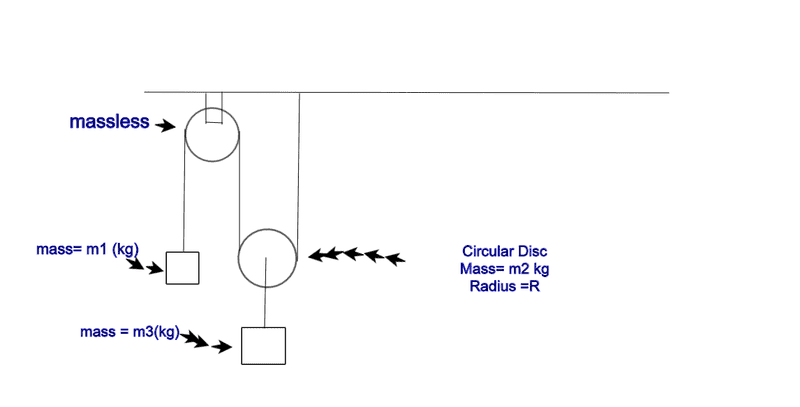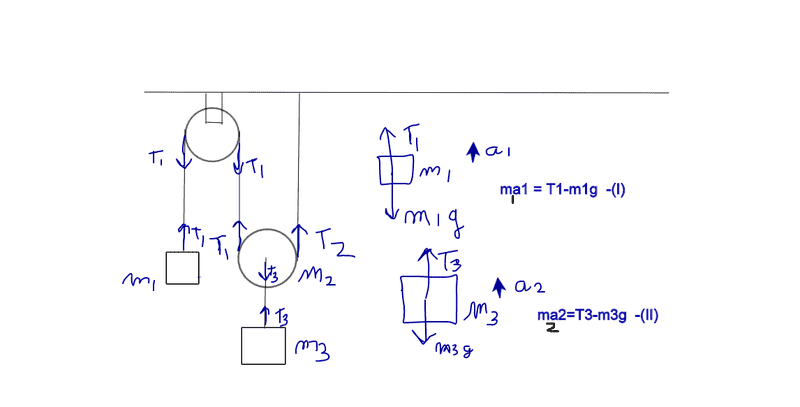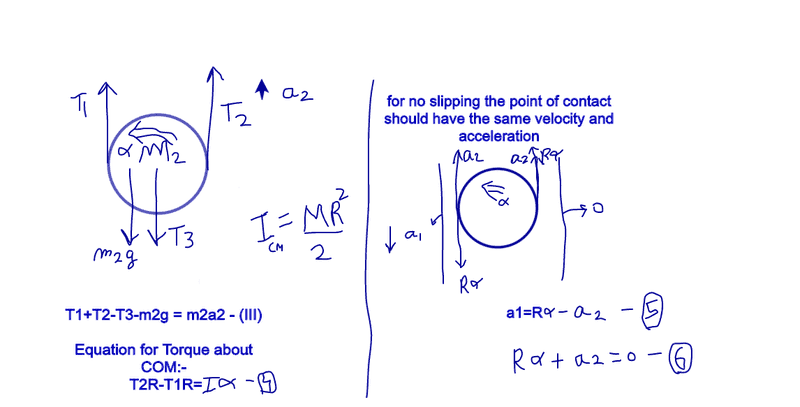# Rotational Dynamics Pulley Problem Help needed

1.) Homework Statement
A Pulley System is shown below
Find the accelerations of m1, m2 and m3 (such that there is no slipping between the disk and the rope.)
Assume the threads to be massless.## Homework Equations

The Relevant equations i think are newtons 2nd law of motion for translation of m1 and m3

And Rotational as well as Translational equations for m2.
Also Torque about Fixed Axis Of Rotation can be written for m2.

## The Attempt at a Solution

[/B]I have attempted the problem like this :-

assumed m1 to go up with acceleration a1,
assumed m2 and m3 both to go up with acceleration a2.
assumed α to be the angular acceleration of the disk anti clockwise.
assumed tensions T1,T2,T3.
T1 is not equal to T2 as the disk has mass.The other equations are :-The Variable are a1, a2 , T1, T2 , T3, α
6 equations and 6 variables, so it can be solved.

My Question is that are these equations correct and how do i know that my assumed accelerations are in the correct direction??

Please Reply! Thanks!haruspex
Homework Helper
Gold Member
In equation (II) there is a handwritten subscript 2 that should be 3.
All else looks good. Are you stuck, or just wanted a progress check?

•Sahil Kukreja and bznm
Will the answer depend on the direction of angular acceleration or it will come out to be same?

In equation (II) there is a handwritten subscript 2 that should be 3.
All else looks good. Are you stuck, or just wanted a progress check?

thanks, yes thats a typo.
I wanted to know if my direction of accelerations and angular acceleration are correct or not?

haruspex
Homework Helper
Gold Member
thanks, yes thats a typo.
I wanted to know if my direction of accelerations and angular acceleration are correct or not?
I cannot tell since you have not posted your solution of the equations.
The directions you choose for the variables at the start is immaterial. All you do there is choose which direction is positive for that variable. If it turns out that the accekeration is in that direction you will get a positive answer. If it is the other way you will get a negative answer.
There are generally three approaches:
- In the "naive" approach, you make a genuine guess at which way each object will accelerate and pick the positive directions accordingly;
- in what one might call the Cartesian approach, you stick to a convention like up is positive and right is positive. But in general some accelerations could be at an angle, so you then have to break it into components.
- Assign the positive directions at random.
Or you could use a hybrid.
Whichever you choose, the important thing is to be consistent through the equations.